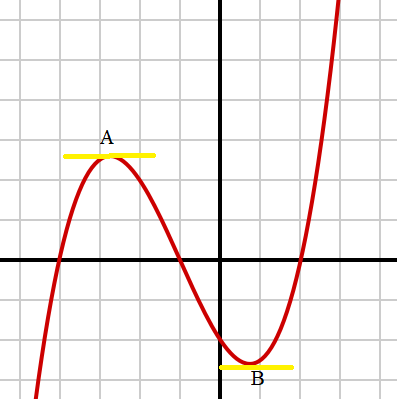# nth Derivative: Definition & Formula ExamplesA derivative is the slope of a tangent line at a point. Here, the derivatives at points A and B are zero.The nth derivative refers to any one of a number of higher order derivatives of a function

When you take the derivative of function one time, you get the first derivative.  Differentiating the new function another time gives you the second derivative.  Likewise, a third, fourth or fifth application of the rules of differentiation gives us the third derivative, fourth derivative and fifth derivative, respectively.  The nth derivative is a formula for all successive derivatives of a function.

## Examples: Finding The nth Derivative

Watch the video for three examples:

Finding the nth derivative means to take a few derivatives (1st, 2nd, 3rd…) and look for a pattern. If one exists, then you have a formula for the nth derivative. To find the nth derivative, find the first few derivatives to identify the pattern.  Apply the usual rules of differentiation to a function, then find each successive derivative to arrive at the nth

Example 1: Find the nth derivative of f(x) = xn

Since this function has exponents, use the Power Rule to find the first few derivatives.  Once you calculate the first 3-4 derivatives of the function, you should get a sense of the overall pattern.

The first three derivatives of this function are:

1. f′(x) = nxn – 1
2. f′′(x) = n(n – 1)xn – 2
3. f′′′(x) = n(n – 1) (n – 2)xn – 3

A pattern is emerging: Each successive derivative adds another layer of n minus (n -), which can be written as:

n(n-1) (n-2)…(n – n + 1)

You can write the formula for the nth derivative as:

f(n) = n(n – 1) (n – 2)…(n – n + 1)xn – n

The pattern of successive multiplication is called a factorial and is written as n!.

Example 2: Find the nth derivative of f(x) = 1/x

Find the first three derivatives of the function and then solve:

1. f′(x) = -1/x2
2. f′′(x) = 1 ∙ 2/x3
3. f′′′(x) = 1 ∙ 2 ∙ 3/x4

The pattern emerging involves adding an additional consecutive number to the numerator and another exponent to the denominator.  We can write the nth derivative as:

f(n) = (-1)n ∙ n!/xn + 1

## References

Orloff, J. Differentiation.

CITE THIS AS:
Stephanie Glen. "nth Derivative: Definition & Formula Examples" From StatisticsHowTo.com: Elementary Statistics for the rest of us! https://www.statisticshowto.com/derivatives/nth-derivative-definition/